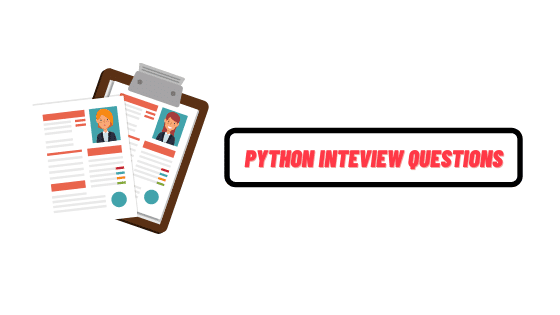This book covers the python interview questions with answers. so if you are preparing for python developer interview then this pdf book notes is for you.

### Book contents

1. What is python and its benefits
2. What is PEP 8
3. What is pickling and unpickling
4. How python is interpreted
5. How memory is managed in python
6. What are the tools that help to find bugs or perform static analysis
7. What are Python decorators
8. What is the difference between list and tuple
9. How are arguments passed by value or by reference
10. What is Dict and List comprehensions are
11. What are the built-in type does python provides
12. What is namespace in python
13. What is lambda in python
14. Why lambda forms in python do not have statements
15. What is a pass in python
16. what are iterators
17. What is a unit test in python
18. What is Slicing
19. What are generators
20. What is docstring
21. How can you copy an object in python
22. What is a negative index in python
23. How you can convert a number to a string
24. What is the difference between Xrange and range
25. What are module and package
26. What are the rules for local and global variables
27. How can you share global variables across modules
28. Explain how can you make a python script executable on Unix
29. Explain how to delete a file in python
30. Explain how can you generate random numbers in python
31. Explain how can you access a module written in python from c
32. Mention the use of // operator in python
33. Mention five benefits of using python
34. Mention the use of the split function
35. Explain what is a flask and it's benefits
36. What is the difference between Django, Pyramid, and flask
37. What is flask-WTF and it's features
38. Explain what is the common way for the flask script to work
39. Explain how you can access session in the flask
40. Is flask an MVC model and if yes give an example showing MVC pattern for your application